Next: Ensemble learning Up: Posterior approximations Previous: Laplace approximation   Contents

## EM algorithm

The EM algorithm was originally presented by Dempster et al. in 1977 . They presented it as a method of doing maximum likelihood estimation for incomplete data. Their work formalised and generalised several old ideas. For example Baum et al.  used very similar methods with hidden Markov models several years earlier.

The term incomplete data'' refers to a case where the complete data consists of two parts, only one of which is observed. Missing part can only be inferred based on how it affects the observed part. A typical example would be the generative model in Section 3.1.1, where the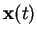are the observed data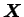and the sources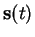are the missing data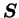.

The same algorithm can be easily interpreted in the Bayesian framework. Let us assume that we are trying to estimate the posterior probability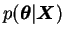for some model parameters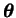. In such a case, the Bayesian version gives a point estimate for posterior maximum of.

The algorithm starts at some initial estimate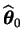. The estimate is improved iteratively by first computing the conditional probability distribution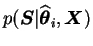of the missing data given the current estimate of the parameters. After this, a new estimate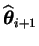for the parameters is computed by maximising the expectation ofover the previously calculated. The first step is called the expectation step (E-step) and the latter is called maximisation step (M-step) [16,57].

An important feature in the EM algorithm is that it gives the complete probability distribution for the missing data and uses point estimates only for the model parameters. It should therefore give better results than simple MAP estimation.

Neal and Hinton showed in  that the EM algorithm can be interpreted as minimisation of the cost function given by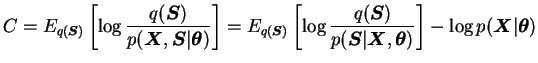(3.10)

where at step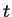,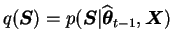. The notation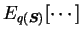means that the expectation is taken over the distribution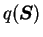. This interpretation can be used to justify many interesting variants of the algorithm.Next: Ensemble learning Up: Posterior approximations Previous: Laplace approximation   Contents
Antti Honkela 2001-05-30